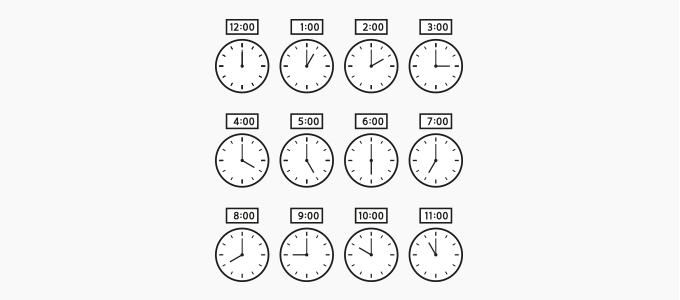Consecutive Numbers - Definition with Examples

The Complete K-5 Math Learning Program Built for Your Child

• 30 Million Kids

Loved by kids and parent worldwide

• 50,000 Schools

Trusted by teachers across schools

• Comprehensive Curriculum

Aligned to Common Core

What is Consecutive Numbers?

Numbers that follow each other continuously in the order from smallest to largest are called consecutive numbers.

For example:

1, 2, 3, 4, 5, 6, and so on are consecutive numbers.Consecutive Even Numbers:

Even numbers are numbers that end with 0, 2, 4, 6 or 8. The examples of consecutive even numbers are:

0, 2, 4, 6, 8, 10, 12, ....

Consecutive Odd Numbers:

Odd numbers are numbers that end with 1, 3, 5, 7 or 9. The examples of consecutive odd numbers are:

1, 3, 5, 7, 9, 11, 13, 15, ....

Consecutive Even and Odd Integers:

We can also have consecutive even and odd integers.

Example: Consecutive Even Integers:  – 8, –6, –4, –2, 0, 2, 4, 6, .....

Example: Consecutive Odd Integers: –9, –7, –5, –3, –1, 1, 3, 5, 7, ....

The term consecutive numbers is often used to frame word problems.

Example: The sum of two consecutive numbers is 55. What are the numbers?

Here, let the first number be a. Since the numbers are consecutive, the other number will be a + 1

We now form an equation as per the given information:

Sum of the numbers = 55 =  a + a + 1

We should choose the numbers such that their sum is 55.

26 is nearly half of 55.

Let the two number be 26 and 27;

26 + 27 = 53   ✘

So, the two numbers are not 26 and 27.

Let us choose the next number, 28. So, the two numbers are 27 and 28.

27 + 28 = 55 ✓

Therefore, the numbers are 27 and 28.

Example: The product of two consecutive odd numbers is 143. What are the numbers?

We should choose the numbers whose product is nearly 143.

We know that 12 × 12 = 144

But, 12 is an even number.

Consecutive odd numbers near 12 are 11 and 13.

Let us find their product; 11 × 13 = 143 ✓

Therefore, the numbers are 11 and 13.

 Fun Facts The sum of any two consecutive numbers is always odd. Example, 4 + 5 = 9; –8 + (–7) = –15.

Won Numerous Awards & Honors